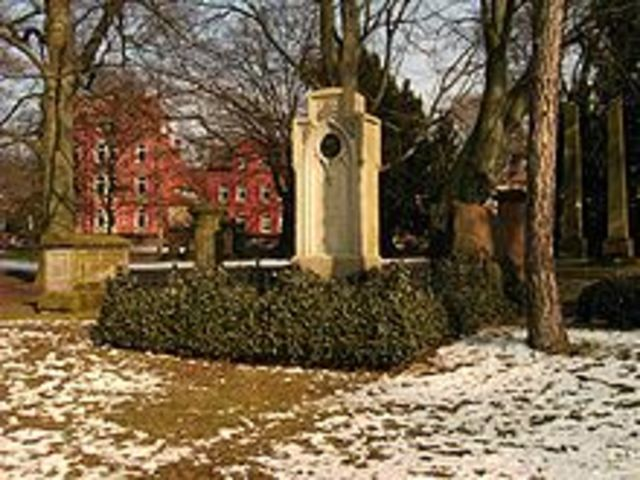• # Date of BirthJohann Carl Friedrich Gauss was born in Brunswick, Germany
• Period: to

• # Modular Arithmetic

The year 1796 was most productive for both Gauss and number theory. He discovered a construction of the heptadecagon on 30 March. He further advanced modular arithmetic, greatly simplifying manipulations in number theory.[citation needed] On 8 April he became the first to prove the quadratic reciprocity law. This remarkably general law allows mathematicians to determine the solvability of any quadratic equation in modular arithmetic.
• # Prime Number Theorem

The prime number theorem, conjectured on 31 May, gives a good understanding of how the prime numbers are distributed among the integers.
• # EYPHKA!

Gauss also discovered that every positive integer is representable as a sum of at most three triangular numbers on 10 July and then jotted down in his diary the note: "ΕΥΡΗΚΑ! num = Δ + Δ + Δ".
• # Finite Fields

On October 1 he published a result on the number of solutions of polynomials with coefficients in finite fields, which 150 years later led to the Weil conjectures.
• # Earned Doctorate

Gauss attended the University of Göttingen from 1795 to 1798. He earned his doctorate in 1799 at the University of Helmstedt.
• # ‘Disquisitiones Arithmeticae’

Carl Gauss published the book ‘Disquisitiones Arithmeticae’ (Arithmetical Investigations) in 1801. He introduced the symbol ‘≡’ for congruence in this book and gave the first two proofs of the law of quadratic reciprocity.